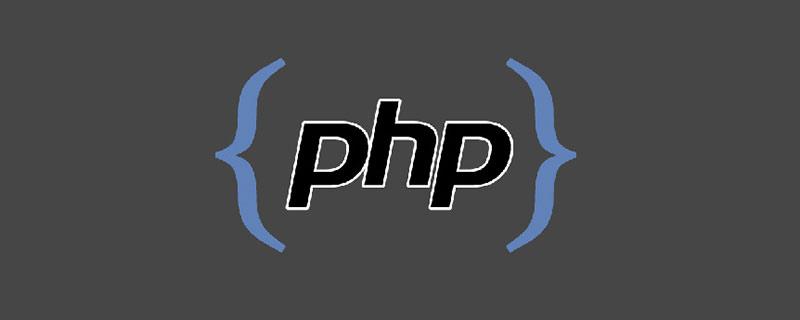# php stdclass如何转数组_编程技术_编程开发技术教程PHP中把stdClass Object转array的几个方法

PHP和JS通讯通常都用json，但用 json 传过来的数组并不是标准的array，而是 stdClass 类型。那么我们可以参考下面的几个方法进行转换。

```//PHP stdClass Object转array
function object_array(\$array) {
if(is_object(\$array)) {
\$array = (array)\$array;
} if(is_array(\$array)) {
foreach(\$array as \$key=>\$value) {
\$array[\$key] = object_array(\$value);
}
}
return \$array;
}```

`\$array = json_decode(json_encode(simplexml_load_string(\$xmlString)),TRUE);`

```function object2array_pre(&\$object) {
if (is_object(\$object)) {
\$arr = (array)(\$object);
} else {
\$arr = &\$object;
}
if (is_array(\$arr)) {
foreach(\$arr as \$varName => \$varValue){
\$arr[\$varName] = \$this->object2array(\$varValue);
}
}
return \$arr;
}```

```function object2array(&\$object) {
\$object =  json_decode( json_encode( \$object),true);
return  \$object;
}```

php stdclass如何转数组

php百分数如何转小数

## php文件操作系列大汇总（持续更新~）

php文件操作系列大汇总（持续更新~）

## php 转化为两位小数的方法_编程技术_亿码酷站

php 转化为两位小数的方法_编程技术_亿码酷站

php怎么实现登陆后跳转网页

## php如何实现date转string_亿码酷站_亿码酷站

php如何实现date转string_亿码酷站_亿码酷站